Courses

# Extra Questions- Practical Geometry Class 8 Notes | EduRev

## Mathematics (Maths) Class 8

Created by: Full Circle

## Class 8 : Extra Questions- Practical Geometry Class 8 Notes | EduRev

The document Extra Questions- Practical Geometry Class 8 Notes | EduRev is a part of the Class 8 Course Mathematics (Maths) Class 8.
All you need of Class 8 at this link: Class 8

Question 1: Draw a rectangle PQRS in which PQ = 7.2 cm and QR = 5.4 cm.
Solution: Steps of construction: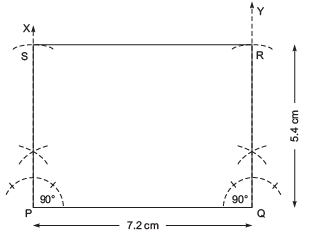I. Draw a line segment PQ = 7.2 cm.
II. At P, draw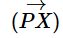such that ∠QPS = 90°.
III. From PX, cut off PS = 5.4 cm.
IV. At Q, draw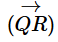such that ∠PQY = 90°.
V. From QY, cut off QR = 5.4 cm.
VI. Join R and S.
Thus, PQRS is the required rectangle.

Question 2: Draw a quadrilateral in which AB = 4.5 cm, BC = 5.1 cm, CD = 3.9 cm, ∠B = 90° and ∠C = 120°.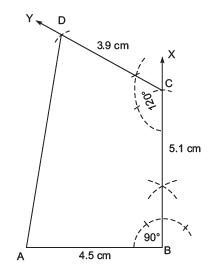Solution: Steps of construction:
I. Draw a line segment AB = 4.5 cm.
II. At B, construct ∠ABX = 90°.
III. From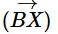cut off BC = 5.1 cm.
IV. AT C, draw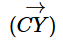such that ∠BCY = 120°.
V. From, cut off CD = 3.9 cm.
Thus, ABCD is the required quadrilateral

Question 3: Draw a square ABCD such that AB = 6.3 cm.
Solution: Steps of construction: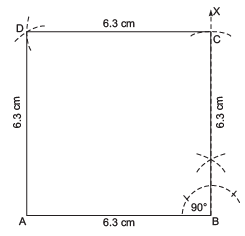I. Draw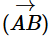= 6.3 cm.
II. At B, draw, such that ∠ABX = 90°.
III. Fromcut off BC = 6.3 cm.
IV. With centre C and radius = 6.3 cm, draw an arc.
V. With centre A and radius = 6.3 cm, draw another arc to intersect the previous arc at D.
VI. Join DA and CD.
Thus, ABCD is the required square.

## Mathematics (Maths) Class 8

217 videos|147 docs|48 tests

,

,

,

,

,

,

,

,

,

,

,

,

,

,

,

,

,

,

,

,

,

;